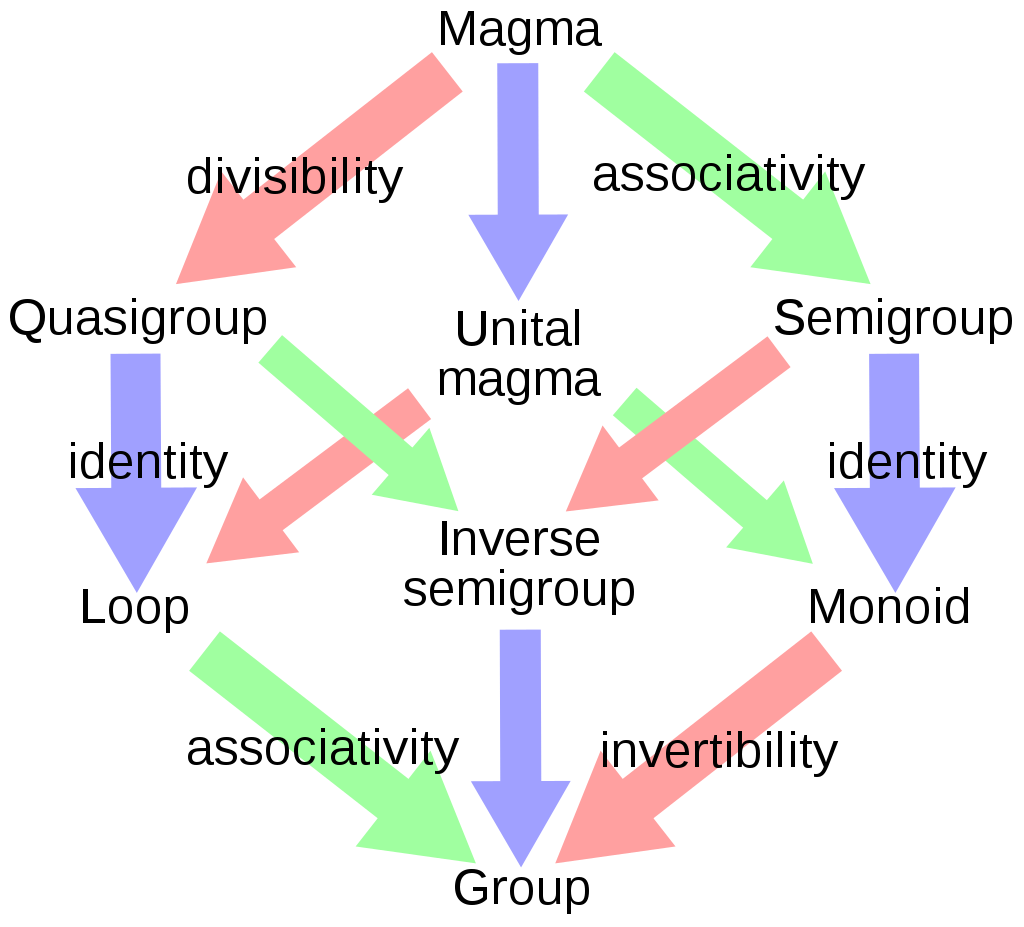## 代数结构

• 内部操作 (internal operation)
$X \times X$$X$ 的映射。
• 外部操作 (external operation)
$A\times X$$X$ 的映射。其中 $A$ 是一个集合。

### 群Fig1：从 Magma (一个集合与一个封闭的内部操作) 到 Group 的代数结构

$X \times X \rightarrow X,\ (x,y) \mapsto xy$

$(xy)z = x(yz),\ \forall x,y,z \in X$

$X \times X \rightarrow X,\ (x,y) \mapsto xy$

• 结合律

$(xy)z = x(yz),\ \forall x,y,z \in X$

• 存在恒元 $e$

$xe = ex = x,\ \forall x \in X$

$X \times X \rightarrow X,\ (x,y) \mapsto xy$

• 结合律：

$(xy)z = x(yz),\ \forall x,y,z \in X$

• 存在恒元 $e$

$xe = ex = x,\ \forall x \in X$

• 存在逆元
$\forall x \in X$，集合 $X$ 中存在一个元素，记为 $x^{−1}$，使得

$x ^{−1} x = xx^{−1} = e$

$f \in \mathrm{Hom}(G_1 ,G_2 ) \Leftrightarrow f(g_1,h_1 ) = f(g_1 )f(h_1 ),\ \forall g_1 ,h_1 \in G_1 .$

$xy \rightarrow x + y$

### 环

$(x,y) \mapsto xy ,\ (x,y) \mapsto x + y$

• 对于加法，$X$ 是一个阿贝尔群。
• 乘法要求满足结合律 (成半群)，且满足相对于加法的分配律：
$\forall x,y,z \in X$，有：

\begin{aligned} &(xy)z = x(yz) \\ &x(y + z) = xy + xz \\ &(y + z)x = yx + zx \\ \end{aligned}

• 阿贝尔环 (abelian ring)
乘法可交换

• 幺环 (ring with identity)
（乘法成幺半群）存在乘法恒元，即存在 $e \in X$，使得

$xe = ex = x,\ \forall x \in X$

$X$ 是一个幺环，元素 $x\in X$ 有逆（对于乘法），则称元素 $x$可逆的 (regular, invertible, non singular)

### 模

$R$ 上的模 $X$ 为一个阿贝尔群，以及一个称为标量乘积的外部操作：

$R \times X \rightarrow X,\ (\alpha,x) \mapsto \alpha x$

\begin{aligned} &\alpha (x + y) = \alpha x + \alpha y\\ &(\alpha + \beta)x = \alpha x + \beta x\\ &(\alpha\beta)x = \alpha(\beta x)\\ \end{aligned}

（若 $R$ 有恒元，还要求标量乘积满足：$ex = x,\ \forall x \in X$

• $R$ 中有两个内部操作：环的加法和乘法。
• $X$ 自身还有一个内部的操作：阿贝尔群的加法。
• 将这些内部操作联系起来的外部操作：标量乘积。

### 代数

• $X$ 自身形成一个环
• 外部操作 $R \times X \rightarrow X,\ (\alpha,x) \mapsto \alpha x$ 满足:

$\alpha(xy) = (\alpha x)y = x(\alpha y)$

• $R$ 上的乘法和加法。
• $X$ 上的乘法和加法。
• 将这些内部操作联系起来的外部操作：标量乘积。